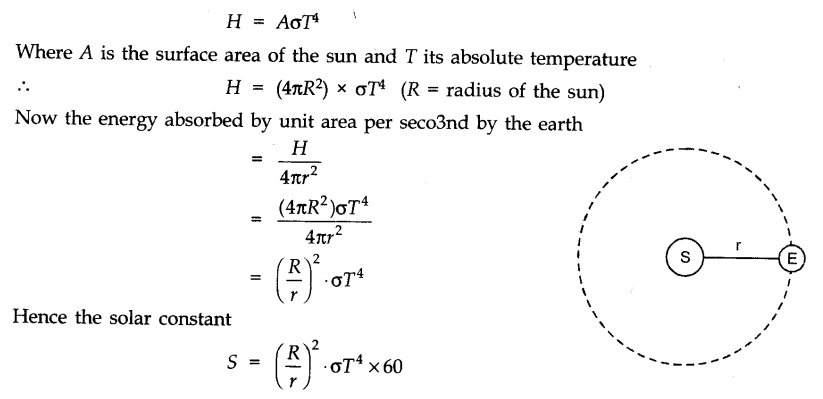# Thermal Properties of Matter

Heat is the form of energy transferred between two (or more) systems or a system and its surroundings by virtue of temperature difference.

The SI unit of heat energy transferred is expressed in joule (J).
In CGS system, unit of heat is calorie and kilocalorie (kcal).

1 cal = 4.186 J and 1 kcal = 1000 cal = 4186 J.

ntent -->

Temperature of a substance is a physical quantity which measures the degree of hotness or coldness of the substance. The SI unit of temperature is kelvin (K) and °C is a commonly used unit of temperature.

A branch of science which deals with the measurement of temperature of a substance is known as thermometry. A device used to measure the temperature of a body is called thermometer.

A thermometer calibrated for a temperature scale is used to measure the value of given temperature on that scale. For the measurement of temperature, two fixed reference points are selected.

The two convenient fixed reference points are the ice point and the steam point of water at standard pressure, which are known as freezing point and boiling point of water at standard pressure.

The two familiar temperature scales are the Fahrenheit temperature scale and the Celsius temperature scale. The ice and steam point have values 32°F and 212°F respectively, on the Fahrenheit scale and 0°C and 100°C on the Celsius scale.

On the Fahrenheit scale, there are 180 equal intervals between two reference points, and on the Celsius scale, there are 100.

If tc and tF are temperature values of a body on Celsius temperature scale and Fahrenheit temperature scale respectively, then the relationship between Fahrenheit and Celsius temperature is given by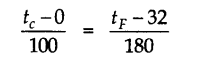An ideal gas obeys the following law. That is PV = gRT, where P,V and T are the pressure, volume and temperature of the gas respectively, g is the number of moles in an ideal gas and R = 8.31 J mol-1 K-1 is known as universal gas constant.

The equation, PV – gRT is known as ideal gas equation.

The absolute minimum temperature for an ideal gas, inferred by extrapolating the straight-line P – T graph is found to be – 273.15 °C and is designated as absolute zero. Absolute temperature scale (T) and Celsius scale are related by
t° C = T – 273.15

## Thermal Expansion

The increase of size of a body due to the increase in the temperature is called thermal expansion. Three types of expansions can take place in solids viz. linear, superficial and volume expansion,

## Ø  Linear Expansion:

The increase in the length of a solid on heating is called linear expansion.
If the temperature of a rod of original length l is raised by a small amount Δt, its length increases by Δl. Then the linear expansion is given by
Δl = l ∞ Δt
where a is the coefficient of linear expansion of the given solid. The unit of α is per degree Celsius (°C-1) in the CGS and per kelvin (K-1) in the SI system.

## Ø  Superficial or Area Expansion:

The increase in surface area of the solid on heating is called superficial expansion.
If A0 is the area of a solid at 0°C and A (its area at t°C then At = A0(l + βt)
where β is known as the coefficient of superficial expansion. Unit of β is °C-1 or K-1.

Also Checkout:  Waves

## Ø  Volume Expansion:

The increase in volume of the solid on heating is called volume expansion.
The change in the volume of a solid with a change in temperature Δt is given by Δv = Vγ Δt
where y is the coefficient of volume expansion.

The relation among coefficients of linear expansion (α), superficial expansion (β) and volume expansion (γ) is given as

For a given solid, the three coefficients of expansion α  , β, γ are not constant. Their values depend on the temperature range.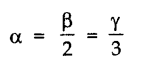Liquids have volume expansion only. If we do not take into account the expansion of solid container, then the expansion of liquid is called apparent expansion.

On the other hand, if we take into account the expansion of solid too, it is referred as the real expansion of liquid. It is found that γr = γa + γg, where γr= real expansion coefficient of liquid, γa = apparent expansion coefficient of liquid and γg = volume expansion coefficient of container vessel (glass).

Water exhibits an anomalous behavior. It contracts on heating between 0 °C and 4 °C but expands on heating beyond 4 °C. Thus, specific volume of water is minimum at 4 °C or density of water is maximum at 4 °C. This property of water has an important environmental effect.

## Thermal Stress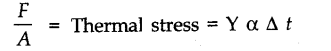When a rod is held between two fixed supports and its temperature is increased, the fixed supports do not allow the rod to expand, which results in a stress which is called thermal stress.
Thermal stress in the rod is given by

where Y is the Young’s modulus for the material of the rod, A is the area cross-section of the rod, a is the coefficient of linear expansion and F is the developed force in the rod.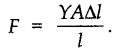## Thermal Capacity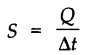The thermal capacity of a body is the quantity of heat required to raise the temperature of the whole of the body through a unit degree. It is measured in calorie per °C or joule per K.
If Q be the amount of heat needed to produce a change in temperature (Δt) of the substance, then thermal capacity of the substance is given by

Dimensional formula of heat capacity is [ML2T -2K-1 ],\

## Specific Heat Capacity

The specific heat capacity (also referred to as specific heat) of a substance is the amount of heat required to raise the temperature of a unit mass of substance through 1 °C. It is measured in cal g-1(°C)-1 or J kg-1 K-1.
The specific heat capacity of a substance is given by

where m is mass of substance and Q is the heat required to change its temperature Δt.
Molar specific heat capacity of a substance is defined as the amount of heat required to raise the temperature of 1 mole of the substance by 1°C.
It is given by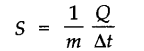The unit of molar specific heat capacity is J mole-1 K-1  in SI system and Cal mol-1 °C-1 in CGS system.
The dimensional formula of molar specific heat capacity is [ML2T-2 K-1 mole-1].
• Calorimetry
Calorimetry is concerned with the measurement of heat, the basic apparatus for this purpose being called the calorimeter.
When two bodies at different temperatures are ‘mixed’, heat ‘flows’ from the body at a higher temperature to the one at a lower temperature, until a common ‘equilibrium’ temperature is reached. Assuming this ‘heat exchange’ to be confined to the two bodies alone (i.e, neglecting any heat loss to the surroundings) we have, from the law of energy conservation:
Heat gained by one body = heat lost by the other.
• Transition of matter from one state (solid, liquid and gas) to another is called a change of state.
• The change of state from solid to liquid is called melting and from liquid to solid is called fusion. It is observed that the temperature remains constant until the entire amount of the solid substance melts i.e., both the solid and liquid states of the substance co-exist in thermal equilibrium during the change of state from solid to liquid.
• The temperature at which a solid melts is called its melting point. The value of melting point of a solid is characteristic of the substance and depends on pressure also.
• Melting of ice under increased pressure and refreezing on reducing the pressure is called regelation.
• The change of state from liquid to vapour (or gas) is called vaporisation. The temperature at which the liquid and vapour states of a substance co-exist is called its boiling point.
• The change from solid state to vapour state without passing through the liquid state is called sublimation.
• The Basic Heat Formula
The heat Q required to raise the temperature of a mass m of a substance of specific heat capacity s through t degrees is given by

Also Checkout:  Light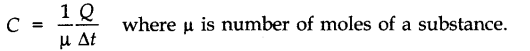i.e., Heat required = mass x specific heat x change in temperature

• Latent Heat
The latent heat of a substance is the amount of heat energy required to change the state of unit mass of the substance from solid to liquid or from liquid to gas/vapour without any change in temperature.
• The latent heat of fusion (Lf) is the heat per unit mass required to change a substance from solid into liquid at the same temperature and pressure. The latent heat of vaporization (Lv) is the heat per unit mass required to change a substance from liquid to vapour state without a change in temperature and pressure.
• Heat Transfer
Heat can be transferred from one place to another by three different methods, namely, conduction, convection and radiation. Conduction usually takes place in solids, convection in liquids and gases, and no medium is required for radiation.
(i) Conduction: According to Maxwell, conduction is the flow of heat through an unequally heated body from places of higher temperature to those of lower temperature. Rate of heat transfer is given by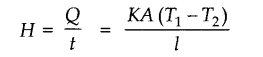where K is called Thermal Conductivity and A is the area of cross-section.
(ii) Convection: Maxwell defines convection as the flow of heat by the motion of the hot body itself carrying its heat with it.
(iii) Radiation: Radiation is the mode of heat transfer in which heat travels directly from one place to another without the agency of any intervening medium.
• Thermal conductivity is defined as heat energy transferred in unit time from unit area having a unit difference in temperature over unit length. It is expressed in Js-1 m-1 °C-1 or W-1 K-1
• Thermal Resistance
The thermal resistance of a body is a measure of its opposition to the flow of heat through it. It is defined as

Also Checkout:  Electromagnetism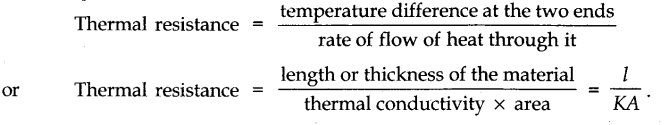## • Newton’s Law of Cooling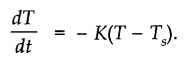Newton’s law of cooling states that the rate of loss of heat of a body is directly proportional to the difference in temperature of the body and the surroundings, provided the difference in temperature is small, not more than 40 °C.

– ve sign implies that as time passes, temperature T decreases.
When an object at temperature T1 is placed in a surrounding of temperature T2 the net energy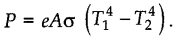## Ø  Emissive Power:

The amount of heat energy rediated per unit area of the surface of a body, per unit time and per unit wavelength range is constant which is called as the ’emissive power’ (eλ) of the given surface, given temperature and wavelength. Its S.I. unit is Js-1 m-2 .

# Ø Absorptive Power:

When any radiation is incident over a surface of a body, a part of it gets reflected, a part of it gets refracted and the rest of it is absorbed by that surface. Therefore, the ‘absorptive power’ of a surface at a given temperature and for a given wavelength is the ratio of the heat energy absorbed by a surface to the total energy incident on it at a certain time. It is represented by (aλ). It has no unit as it is a ratio.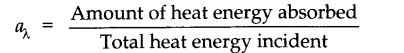## Ø  Perfect Black Body:

A body is said to be a perfect black body is its absorptivity is 1. It neither reflects nor transmits but absorbs all the thermal radiations incident on it irrespetive of their wavelengths.

## Ø  Wein’s Displacement Law:

This law states that as the temperature increases, the maximum value of the radiant energy emitted by the black body, move towards shorter wavelengths. Wein found that “The product of the peak wavelength ( λm) and the Kelvin temperature (T) of the black body should remain constant.”  λm x T= b
Where b is constant known as Wein’s constant. Its value is 2.898 x 10-3 mk.

## Ø  Stefan’s Law: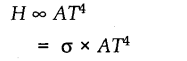This law states that the thermal radiations energy emitted per second from the surface of a black body is directly proportional to its surface area A and to the fourth power of its absolute temperature T.
Emission coefficient or degree of blackness of a body is represented by a dimensionless quantity ε, 0 < ε < 1. If ε = 1 then the body is perfectly black body. Hence

Let us consider an object at absolute temperature T and To be the temperature of the surroundings.

H1 = Rate of energy emitted by the body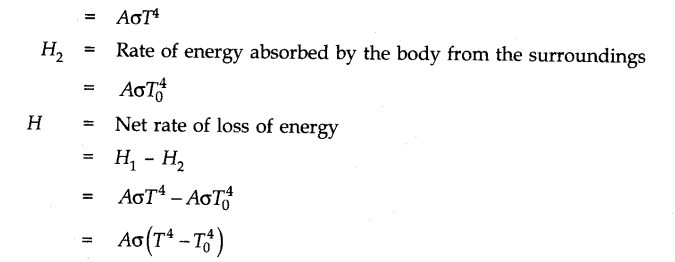## The Solar Constant:

The average energy emitted from the surface of the sun, absorbed per unit area, per minute by the earth is constant which is called as solar constant which is represented by S whose value is 8.135 jm-2 min-1.

Let the earth be moving in a circular path of radius r taking sun as its centre.
Taking sun as perfectly black body, the energy radiated per unit time from the surface of the sun is given by